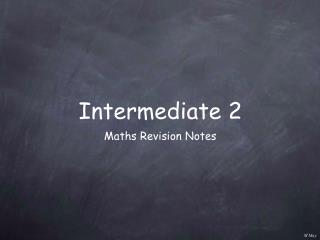DownloadDownload PresentationIntermediate 2

# Intermediate 2

Télécharger la présentation## Intermediate 2

- - - - - - - - - - - - - - - - - - - - - - - - - - - E N D - - - - - - - - - - - - - - - - - - - - - - - - - - -
##### Presentation Transcript

1. Intermediate 2 • Maths Revision Notes

2. Reminder : to _ significant figures to _ decimal places 336.738 = 340 to 2sf 336.738 = 336.7 to 1dp Percentages non-calc calculator interest: simple or compound? 10% = 1/10 171/2% = 0.175 25% = 1/4 eg interest rate = 3% 331/3% = 1/3 new balance = original x 1.03 appreciation increases x 1.06 6% depreciation decreases x 0.94

3. 4πr2h V = 3 4πx7x7x9 3 V = Ah for prism, Volumes A is Area of cross section / base Substitute carefully into formulae Use calculator carefully! (remember brackets) eg r = 7cm h = 9cm show this step V = V = 1847.25648 V = 1850 cm3 correct to 3sf (remember units)

4. arc sector 360 A C Area of rectangle A = l x b A = 1/2 base x ht Area of triangle Area of circle A = πr2 Part of the circumference - arc C = πd Part of the circle area - sector  = = Angle in semicircle = 90˚ Angle tangent / radius = 90˚ Radii equal => isosceles chord cut in half => 90˚ from centre

5. r p q y = sinx • 360˚ y = tanx • • 180˚ 360˚ 360˚ Trigonometry (x, y) r SohCahToa  sin = y/r p/r sinP = cos = x/r q/r cosP = tan= y/x p/q tanP = Pythagoras’ Theorem r2 = p2 + q2 amplitude period

6. B c a A a b c C b sinA sinB sinC b2 + c2 - a2 2bc sinB sinA sinC a c b Area of triangle = 1/2 absinC Sine Rule = = Cosine Rule a2 = b2 + c2 - 2bccosA cosA = = =

7. vertical length horizontal length y2 - y1 x2 - x1 Graph / Equations Plot points from table (x, y) gradient = gradient = m y = mx + c straight line intercept (0, c) ax + by + c = 0 m = y = ax2 + bx + c parabola • equation of best fit line on a scattergraph. simultaneous equations - true at the same time (a, b) x = a y = b • point of intersection of two lines ax + by = c y = px + q ax + b(qx + q) = c • algebraic - by substitution ax + by = c px - by = r (a + p)x = c + r • algebraic - by “adding”

8. 2x2 - 6x - 20 x2 - 25 Algebra multiply out brackets factorise 7(3x + 4) 35x + 15 = 21x + 28 = 5(7x + 3) common factor (2x - 5)(3x + 4) x2 - 81 = 6x2 + 8x - 15x - 20 = (x + 9)(x - 9) = 6x2 - 7x - 20 difference of 2 squares eg Simplify x2 + 9x + 14 = (x + 7)(x + 2) 2x2 - 6x - 20 = 2(x2 - 3x - 10) 2(x - 5)(x + 2) = = 2(x - 5)(x + 2) (x - 5)(x + 5) 2(x + 2) x2 - 25 = (x + 5) = (x - 5)(x + 5)

9. Q2 Q3 Q1 H L _ x circle 1/2/3 of Qs each of 4 groups contains _ no. of favourable P( ) = no. of possible √ Σ(x - x)2 _ s = n - 1 Statistics mean Box plot mode most frequent median middle one in order upper quartile n/4 = _ R _ lower quartile Highest Probability Lowest Range = H - L semi-interquartile range = 1/2(Q3 - Q1) standard deviation => table of values

10. 0 8 5 6 0 5 6 8 0 1 4 5 1 4 0 5 1 1 2 3 6 7 7 2 7 2 6 3 7 2 1 2 3 2 1 3 14 4 • • • • • • • • • • • • • • • • • • • • • Stem and Leaf )( => => n = 14 1| 0 represents 10 ‘things’ Quartiles: = 3 R 2 so Q1 and Q3 Pie Chart Cumulative frequency dot plot symmetrical / skewed toward “tail”

11. Read each question carefully. Show all steps of working. Remember no working = no marks. Leave enough time to attempt all questions. Re-read questions when you finish to make sure you have answered all parts. Having prepared thoroughly, ensure a good night’s sleep before the exam.